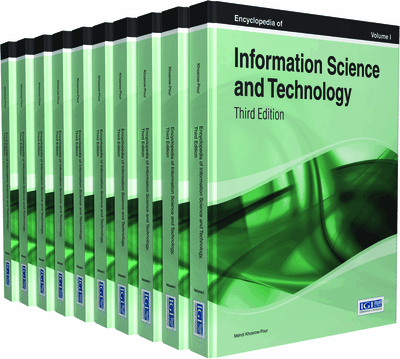# Algorithms for Approximate Bayesian Computation

Tom Burr (Statistical Sciences, Los Alamos National Laboratory, USA) and Alexei Skurikhin (Space Data Systems, Los Alamos National Laboratory, USA)
DOI: 10.4018/978-1-4666-5888-2.ch149
Available
\$29.50
No Current Special Offers

## Abstract

Computer models have many applications in business, medicine, engineering, and science. A typical model has known inputs such as a task schedule, unknown inputs such as the average time to complete a task, and outputs such as product production quality and rates. Our focus is stochastic computer models, which output different results each time the model is run with the same inputs. Such models are calibrated by comparing real data to model predictions. A calibrated computer model can provide a cost-effective option to examine “what-if” questions such as “what if we could reduce the average time to complete a specific task.” This chapter describes an attractive option to calibrate a stochastic computer model that relies not on the raw data but on summary statistics derived from the real data.
Chapter Preview
Top

## Introduction

Computer models can be broadly categorized as deterministic or stochastic. Deterministic models output the same predictions for the same inputs. Stochastic models output different predictions for the same inputs. Our focus is stochastic computer models (SM), and in particular, on a new option to calibrate SMs using approximate Bayesian computation (ABC).

An example used later in this chapter is a SM to model neuronal loss in a region of the human brain that is associated with Parkinson’s disease. Deletion mutations in the mitochondrial DNA (mtDNA) in that brain region are observed to accumulate with age. A deletion mutation converts a healthy copy of mtDNA to the mutant (unhealthy) variant. The number of mutant copies in cases with Parkinson’s disease tends to be higher than in controls without Parkinson’s disease. The role that mtDNA deletions play in neuronal loss is not yet fully understood, so better understanding of how mtDNA deletions accumulate is an area of active research. Henderson et al. (2009) use a simple SM that allows for any of five reactions, occurring at rates to be estimates. The five reactions are mutation, synthesis, degradation, mutant synthesis, and mutant degradation.

Approximate Bayesian computation (ABC) is an approach for using data to calibrate a SM and is especially useful when the likelihood for the data is unknown or intractable. ABC requires a set of summary statistics computed from real data. Then, the same set of summary statistics is computed from the SM for each of many candidate model parameter values. In a parameter acceptance/rejection loop, the candidate SM parameter values that are accepted provide an approximation to the posterior distribution of model parameters given the summary statistics computed from the real data. In a nutshell, ABC favors model parameters for which simulated summary statistics roughly agree with the corresponding summary statistics computed from the observed data. Because ABC relies on user-chosen summary statistics rather than on full data, it becomes computationally feasible. ABC is therefore appealing when the data dimension and/or parameter dimension is large.

This article describes applications of ABC and illustrates the challenges with ABC related to the quality of the approximation to the posterior distribution of model parameters. The challenges involve the fact that the user must choose (1) summary statistics, (2) a distance measure to calculate the distance between summary statistics in the real data and in the model-simulated data, and (3) the acceptance threshold used to accept or reject candidate parameter values in the acceptance/rejection sampling loop.

For a model with parameters θ and data D, a key quantity in Bayesian inference is the posterior distribution of model parameters given by Bayes theorem as

,whereis the probability distribution for θ before observing data D,is the likelihood, andis the marginal probability of the data that is used to normalize the posterior probabilityto integrate to 1 (Aitken, 2010). The likelihoodcan be regarded as the “data model” for a given value of θ. Alternatively, when the data D is considered fixed,is regarded as a function of θ, and non-Bayesian methods such as maximum likelihood find the value of θ that maximizes(Aitken, 2010).

## Key Terms in this Chapter

Approximate Bayesian Computation (ABC): A method that compares summary statistics generated from real data to the same summary statistics generated from the model in order to provide estimates and uncertainty for model parameters.

Partial Posterior Distribution: In Bayesian statistics, a key quantity is estimate the posterior probability density In ABC, the partial posterior distribution Replaces .

Bayesian Calibration of a Computer Model: A method to choose effective model inputs that regards model inputs as unknown and unknowable parameters.

Stochastic Computer Model: A model with known inputs such as a task schedule, unknown inputs such as the average time to complete a task, and stochastic (random) outputs that differ each time the model is run with the same (or different) inputs.

Distance Measure: A measure of closeness (or distance) between two vectors.

Bayes Theorem: A simple theorem that converts one probability distribution (the distribution of the data given the model parameters) to another (the distribution of the model parameters given the data): .

Parameter Acceptance Loop: ABC requires repeated evaluation of the model at many trial input values. Some of the trial input values are accepted during the simulation loop over many trail input values.

Summary Statistics: In the context of ABC, the simulated model outputs are reduced to summary statistics such as means and variances. The vector of summary statistics computed from the real data is compared to the vector of summary statistics computed from the simulated data to evaluate candidate model parameter values.

## Complete Chapter List

Search this Book:
Reset